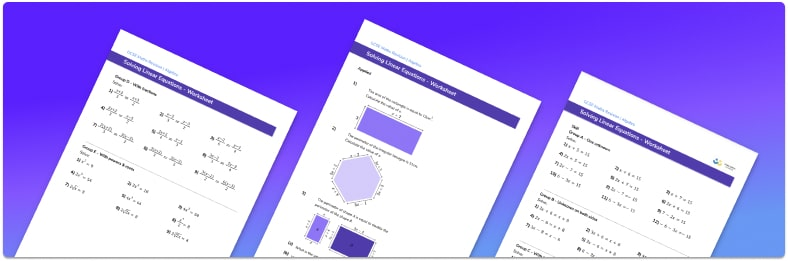# Linear Equations Worksheet• Section 1 of the linear equations worksheet contains 48 skills-based linear equations questions, in 3 groups to support differentiation
• Section 2 contains 3 applied linear equations questions with a mix of worded problems and deeper problem solving questions
• Section 3 contains 3 foundation and higher level GCSE exam style questions
• Answers and a mark scheme for questions are provided
• Questions follow variation theory with plenty of opportunities for students to work independently at their own level
• All questions created by fully qualified expert secondary maths teachers
• Suitable for GCSE maths revision for AQA, OCR and Edexcel exam boards

• This field is for validation purposes and should be left unchanged.

You can unsubscribe at any time (each email we send will contain an easy way to unsubscribe). To find out more about how we use your data, see our privacy policy.

### Linear equations at a glance

Linear equations are equations that contain variables that have powers no higher than one. To solve linear equations the equation needs to be rearranged to make the unknown the subject by using inverse operations. For example if the equation said to add, the inverse of adding is subtracting, if the equations said to multiply the value of the variable by the coefficient, the inverse of multiplying is dividing. Linear equations have one solution that could be a positive or negative integer, a fraction or a decimal.

Solving linear equations can involve one step equations, two step equations or multiple step equations depending on the type of equation. Some linear equations include algebraic fractions containing expressions in their numerator and or denominator, and some equations can also include brackets that can be expanded (using the distributive property of multiplication). Linear equations can sometimes include unknown variables on both sides of the equation which can be solved by first collecting all of the ‘like’ variables so they form one variable on one side of the equal sign.

Understanding how to collect the like terms of an algebraic expression and how to use the order of operations is essential when solving equations.

Looking forward, students can progress with more solving equations worksheets and to additional algebra worksheets, for example a simplifying expressions worksheet, or straight line graphs worksheet.For more teaching and learning support on Algebra our GCSE maths lessons provide step by step support for all GCSE maths concepts.

## Do you have KS4 students who need more focused attention to succeed at GCSE?There will be students in your class who require individual attention to help them succeed in their maths GCSEs. In a class of 30, it’s not always easy to provide.

Help your students feel confident with exam-style questions and the strategies they’ll need to answer them correctly with our dedicated GCSE maths revision programme.

Lessons are selected to provide support where each student needs it most, and specially-trained GCSE maths tutors adapt the pitch and pace of each lesson. This ensures a personalised revision programme that raises grades and boosts confidence.

Find out more